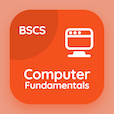Management Online Courses (MBA)

MBA Statistics Quizzes

MBA Statistics Quiz PDF - Complete

# Chow-test Model Quiz Questions Online p. 13

Learn Chow-test Model quiz questions and answers, chow-test model MCQ with answers PDF 13 to learn MBA Statistics online course. Multiple Regression Model trivia questions, chow-test model Multiple Choice Questions (MCQ) for online college degrees. "Chow-test Model Quiz" PDF Book: multicollinearity, f-distribution, chow-test model test prep for 1 year MBA programs.

"Chow test is based on the assumption that the structural break-time period is" MCQ PDF: not known, known, fixed internal, and constant for accredited online MBA programs. Study multiple regression model questions and answers to improve problem solving skills .

## Quiz on Chow-test Model MCQs

MCQ: Chow test is based on the assumption that the structural break-time period is

Known
Not known
Fixed internal
Constant

MCQ: The generated output of F-distribution is always

0
Positive
Negative
Condition-dependent

MCQ: In VIF calculation, if the value of R² is taken as 0, computed VIF would be equal to

1
0
-1
Undefined

MCQ: For data set x equals to 95, 85, 80, 70, 60 and y equals to 85, 95, 70, 70, 65, the standard error is equals to?

8.119
9.237
10.446
11.8196

MCQ: A distribution having a flat degree in terms of kurtosis is known to be

Plety kurtosis
Meso kurtosis
Melto kurtosis
Lefto kurtosis

### Practice Quizzes from Business Statistics Course